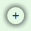# Optimal Price Analysis

The Optimal Price Analysis is a mathematical computation that helps a business identify the point where it realize the maximum of profit.

This Optimal price calculator allows a business to accomplish the following:

• Determine the quantity it needs to produce or sell in order to realize the maximum of profit;
• Determine the selling price it needs to charge for a specific quantity you sell in order to realize the maximum of profit.
 Input Results Variable Cost per Unit: Optimal Price: \$ 0.00 Fixed Cost: Optimal Units: 0.00 Current selling price: Current selling units: Total Revenue: \$ 0.00 Maximum capacity (Units): Total Cost: \$ 0.00 Change to Maximum financing capacity (\$) Price elasticity of demand: Profit: \$ 0.00 Help with Price elasticity of demandShow Current vs. Optimal Note: This calculator is a JavaScript program. You must have JavaScript enabled in order to use it.

## Definitions and terms used in the Optimal Price Analysis

• Variable Cost per Unit: the cost that vary with the production or the purchase of one unit.
• Fixed Cost (FC): the cost that remains constant within a range of production or sales, regardless of the number of units produced or sold within that range. Typical fixed costs are: rent, mortgage, equipment, salaries, insurance, fixed utilities (office utilities) etc.
• Current selling price: the price that a unit is currently sold for.
• Current selling units: the number of units currently sold or produced.
• Maximum capacity (Units): the constraint regarding the maximum number of units that the company can produce or sell.
• Maximum financing capacity: the constraint regarding the financing capacity of the company (bank accounts, credit cards, lines of credit etc.).
• Price elasticity of demand (PeD): the responsiveness of the quantity demanded of a good or service to the increase or decrease in its price. As a general rule, sales increase with drop in prices and decrease with rise in prices.

Typically, PeD has a negative value. For our purpose, in order to make it easier for our users, we will consider the absolute value of PeD. By default we setup PeD as 1 (unit elastic).

The meaning of PeD value is:  Value Meaning PeD = 0 Perfectly inelastic 0 < PeD < 1 Relatively inelastic or inelastic demand PeD = 1 Unit (or unitary) elastic 1 < PeD < ∞ Relatively elastic or elastic demand PeD = ∞ Perfectly elastic
• Optimal Price: the selling price where the company realize its maximum of profit.
• Optimal Units: the number of selling units to be sold in order to realize the maximum of profit.
• Total Variable Cost (VC): the cost that varies directly with the number of units produced or sold. Typical variable costs are: materials, packaging and shipping, sales commission, hourly wages, variable utilities (factory utilities) etc.

Total Variable Cost = Selling Units x Variable Cost per Unit
• Total Cost (TC): total expenses incurred in the process of producing or selling a number of units.

Total Cost (TC) = Fixed Cost (FC) + Total Variable Cost (VC)
• Total Revenue: the total sales value of the units produced or sold.

Total Revenue = Selling Units x Selling Price per Unit
• Profit: the benefits from producing or selling a number of units.

Profit = Total Revenue - Total Cost